### Home > CALC > Chapter 6 > Lesson 6.2.1 > Problem6-66

6-66.
1. A flag is shown below. The curved portion is a quarter-circle. Homework Help ✎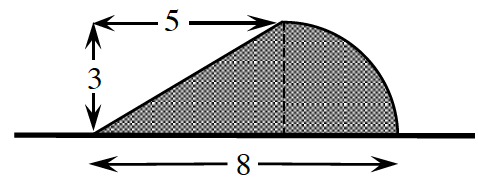1. Imagine rotating the flag about its pole and describe the resulting three-dimensional figure. Draw a picture of this figure on your paper.

2. Find the volume of the rotated flag.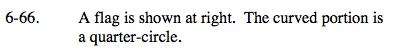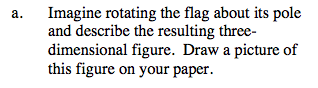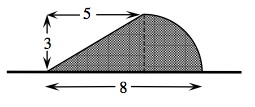The flag would form a hemisphere on top of a cone, is it was rotated.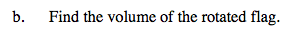$\text{Volume of a Cone }= \frac{1}{3}\pi r^{2}h$

$\text{Volume of a Hemisphere }= \left (\frac{1}{2} \right )\left (\frac{4}{3} \pi r^{3} \right )$